# Mark Willis

•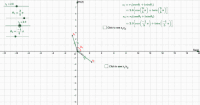### Multiplication and division of complex numbers in modulus-argument form.

Activity

Mark Willis

•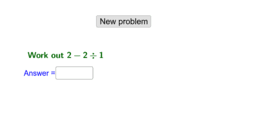### Negative numbers subtraction and division

Activity

Mark Willis

•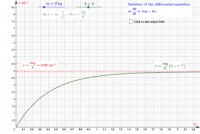### Solution of a 1st order differential equation with slope field.

Activity

Mark Willis

•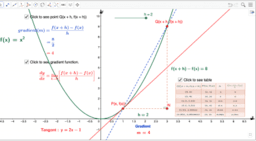### GeoGebra Apps for A-Level Pure Mathematics

Book

Mark Willis

•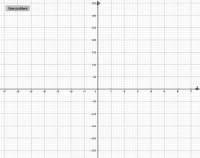### Finding the roots of a cubic using the factor theorem

Activity

Mark Willis

•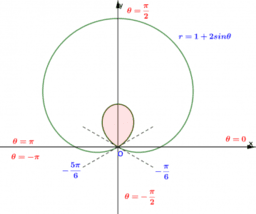### GeoGebra Apps for A-Level Further Pure Mathematics

Book

Mark Willis

•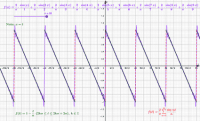### Fourier series for a periodic function 01

Activity

Mark Willis

•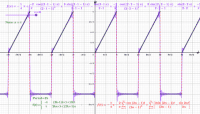### Fourier series for a piecewise periodic function 01

Activity

Mark Willis

•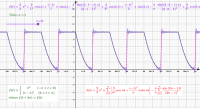### Fourier series for a piecewise periodic function 05

Activity

Mark Willis

•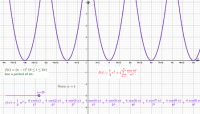### Fourier series for a periodic function 04

Activity

Mark Willis

•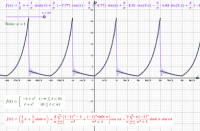### Fourier series for a piecewise periodic function 04

Activity

Mark Willis

•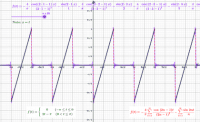### Fourier series for a piecewise periodic function 03

Activity

Mark Willis

•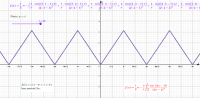### Fourier series for a periodic function 03

Activity

Mark Willis

•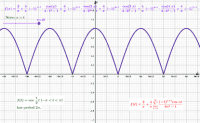### Fourier series for a periodic function 02

Activity

Mark Willis

•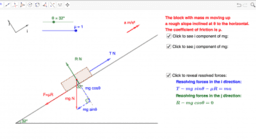### GeoGebra Apps for A-Level Mechanics including FM

Book

Mark Willis

•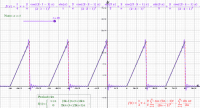### Fourier series for a piecewise periodic function 02

Activity

Mark Willis

•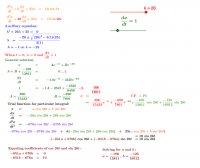### Non-homogeneous 2nd order differential equations

Activity

Mark Willis

•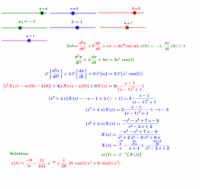### Solving 2nd-order differential equations using Laplace with graph

Activity

Mark Willis

•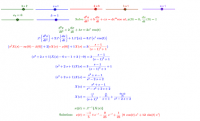### Solving a 2nd-order differential equation using Laplace transformations

Activity

Mark Willis

•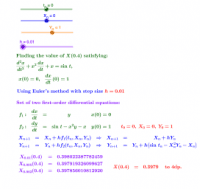### Numerical solution of a second-order differential equation

Activity

Mark Willis

•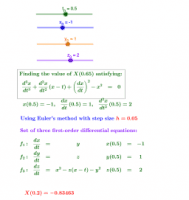### Numerical solution of a third-order differential equation 02

Activity

Mark Willis

•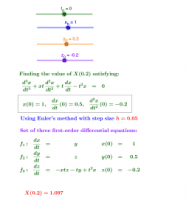### Numerical solution of a third-order differential equation 01

Activity

Mark Willis

•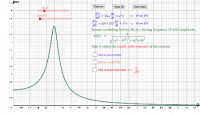### The steady state response of a damped second-order system

Activity

Mark Willis

•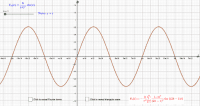Activity

Mark Willis